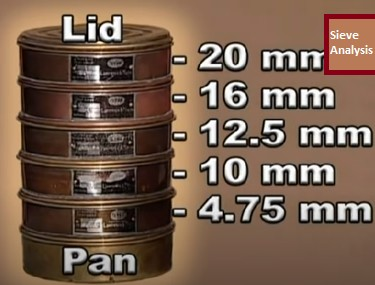# Fineness Modulus of Aggregate Calculator

## Fineness Modulus of Aggregate Calculator – IS### Input:

a) Total Weight of Coarse/Fine Aggregate Kg

*Input values in Column A & B Then Click Calculate below
A) IS Sieve (mm) B) Weight retained (Kg) C) Total Weight Retained (Kg) D) Percentage Weight Retained(%)
Sum: Sum:
Fineness Modulus:

## How to calculate the Fineness Modulus of Aggregate?

Read Here – With Numericals – How to Calculate Fineness modulus of aggregate| Formula with Numerical

## How to Use this Tool?

• Input the weight of fine or coarse aggregate above the table.
• Fill the data in Column A and Column B
• Finally, click on the calculate button to get the fineness modulus of the aggregate.
• Enjoy the calculator.